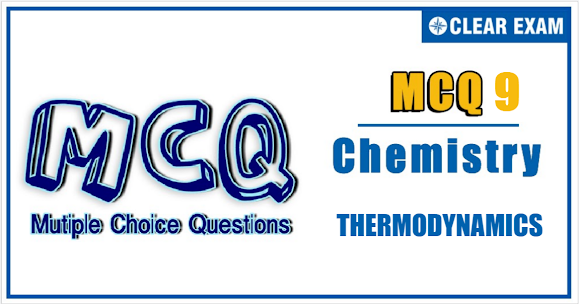## CHEMISTRY THERMODYNAMICS QUIZ-9

IIT JEE exam which consists of JEE Main and JEE Advanced is one of the most important entrance exams for engineering aspirants. The exam is held for candidates who are aspiring to pursue a career in the field of engineering and technical studies.
Chemistry is important because everything you do is chemistry! Even your body is made of chemicals. Chemical reactions occur when you breathe, eat, or just sit there reading. All matter is made of chemicals, so the importance of chemistry is that it's the study of everything.
.

Q1.For an ideal gas undergoing isothermal irreversible expansion:
•  ∆U = 0
•  ∆H = 0
•  ∆S = 0
•   w = 0
Solution
(a,b)
In isothermal process, ∆T = 0 and ∆U = ∆H = 0

Q2.The enthalpy of formation of UF(g) is 22 kcal mol-1 and that of U(g) is 128 kcal mol-1. The bond energy of the F—F bond is 37.0 kcal mol-1. The bond dissociation energy of UF(g) is/are :
•  124.5 kcal mol-1
•  131.1 kcal mol-1
•  521 kcal mol-1
•  623 kcal mol-1
Solution
124.5 kcal mol-1
Q3.The correct relationship is/are :
•  d(∆H)/dT = ∆CP
•  (∆H2 - ∆H1)/∆T = ∆CP
•  ∆H= ∆U + ∆nRT
•  qrev/T = ∆S
Solution
(a,b,c,d)

Q4.Which is not the correct relationship?
•  [dH/dT]P - [dU/dT]T = (+ve)
•  [dU/dV]T = 0 (for ideal gas)
•  [dV/dT]P = nR/P = (for ideal gas)
•  All of these
Solution
(a,c)
[∂H/∂T]P - [∂U/∂T]V = R

Q5.The intensive property/properties is/are
•  Temperature
•  Pressure
•  Internal Energy
•  Heat Capacity
Solution
(a,b)

Q6.For gaseous reactions, if ∆H is the change in enthalpy and ∆U that in internal energy, then
•  ∆H is always greater than ∆U
•  ∆H is always less than ∆U
• ∆H < ∆U only if the number of mole of the products is less than that of the reactants
•  ∆U < ∆H only if the number of mole of the reactants is less than that of the products
Solution
As ΔH = ΔU + ΔngRT
ΔH value is less than or greater than ΔU depending on the value of
Δng which is the change in number of moles of the gaseous components.
As result, (a) and (b) cannot be true.
ΔH < ΔU when Δng < 0
Hence, (d) is the correct answer

Q7.Select the correct statement
•  There is a natural asymmetry between converting work to heat and converting heat to work
•  No process is possible in which the sole result is the absorption of heat from a reservoir and its complete conversion into work
•  For every chemical reaction at equilibrium, standard change in Gibbs free energy is zero
•  At constant temperature and pressure, chemical reactions are spontaneous in the direction of decreasing Gibbs energy
Solution
(b,d)
∆G = -ve for spontaneous process and ∆G ≠ 0 at equilibrium. Statement (b) represents Carnot theorem

Q8.Three identical adiabatic containers have helium, neon and oxygen gases at the same pressure. The gases are compressed to half their original volume. Under these conditions
•  The final temperature of both helium and neon is same
•  The final pressure of the gas in each container is same
•  The final temperature of the gas in each container is same
•  The final pressure of both helium and neon is same
Solution
(a,d)
pVγ = constant and pVγ-1 = constant
Helium and neon are monoatomic gases (γ=1.67),while oxygen is
diatomic gas (γ=1.4). Therefore, final temperature and pressure of both helium and neon are same

Q9.Which is intensive property?
•  Mass
•  Mass/volume
•  Volume
•  Volume/Mass
Solution
(b,d)
Intensive property does not depend upon the mass of substance
Q10. In which of the following entropy increases?
•  Rusting of iron
•  Melting of ice
•  Crystallization of sugar from solution
•  Vaporization of camphor
Solution
(a,b,d)
In conversion of solid ice to liquid and in rusting, the entropy increases#### Written by: AUTHORNAME

AUTHORDESCRIPTION## Want to know more

Please fill in the details below:

## Latest NEET Articles\$type=three\$c=3\$author=hide\$comment=hide\$rm=hide\$date=hide\$snippet=hide

Name

ltr
item
BEST NEET COACHING CENTER | BEST IIT JEE COACHING INSTITUTE | BEST NEET & IIT JEE COACHING: THERMODYNAMICS Quiz-9
THERMODYNAMICS Quiz-9
https://1.bp.blogspot.com/-lkCdUMI0gRs/YN7kqnmD0mI/AAAAAAAAQMU/-E9CdV8zXcsKsPt4ko4vrV8AXzWNOhSFACLcBGAsYHQ/w579-h309/Quiz%2B9.PNG
https://1.bp.blogspot.com/-lkCdUMI0gRs/YN7kqnmD0mI/AAAAAAAAQMU/-E9CdV8zXcsKsPt4ko4vrV8AXzWNOhSFACLcBGAsYHQ/s72-w579-c-h309/Quiz%2B9.PNG
BEST NEET COACHING CENTER | BEST IIT JEE COACHING INSTITUTE | BEST NEET & IIT JEE COACHING
https://www.cleariitmedical.com/2021/07/thermodynamics-quiz-9.html
https://www.cleariitmedical.com/
https://www.cleariitmedical.com/
https://www.cleariitmedical.com/2021/07/thermodynamics-quiz-9.html
true
7783647550433378923
UTF-8

STAY CONNECTED